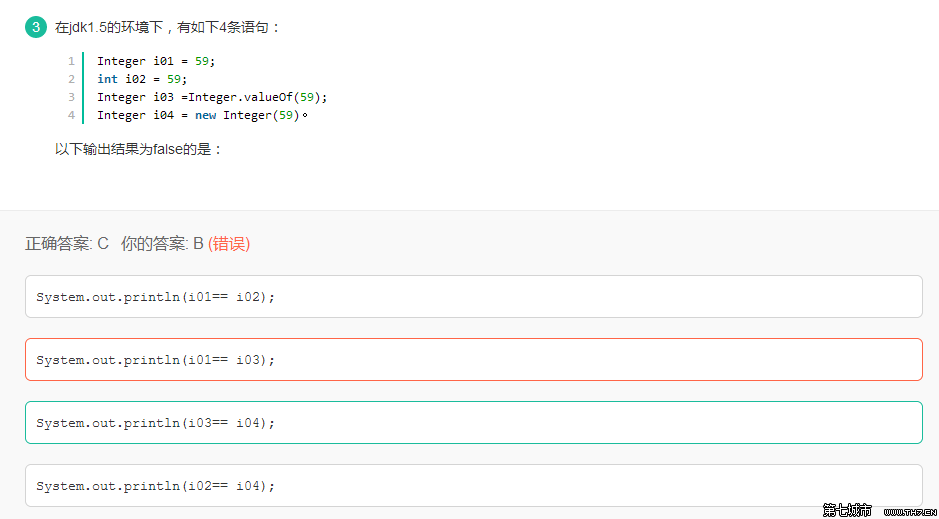# Integer 类型与 int 的==比较

## 困惑的地方

package com.lizi.basic;

public class IntegerDemo {

public static void main(String[] args) {
Integer a = new Integer(3);
Integer b = 3;
int c = 3;

System.out.println(a == b);
System.out.println(a == c);

Integer f1 = 100, f2 = 100, f3 = 150, f4 = 150;
System.out.println(f1 == f2);
System.out.println(f3 == f4);
}

}

false
true
true
false

## Java 数据两种类型 – 基本类型的应用类型

• 基本类型
• 基本数据类类型存的是数值本身
• 引用类型
• 引用类型变量在内存放的是数据的引用

## Integer 用 == 比较的时候往往非常容易出错

        Integer f1 = 100, f2 = 100, f3 = 150, f4 = 150;
System.out.println(f1 == f2);
System.out.println(f3 == f4);

Integer f1 = Integer.valueOf(100);
Integer f2 = Integer.valueOf(100);
Integer f3 = Integer.valueOf(150);
Integer f4 = Integer.valueOf(150); 

    public static Integer valueOf(int i) {
if (i >= IntegerCache.low && i <= IntegerCache.high)
return IntegerCache.cache[i + (-IntegerCache.low)];
return new Integer(i);
}

  cache = new Integer[(high - low) + 1];
int j = low;
for(int k = 0; k < cache.length; k++)
cache[k] = new Integer(j++);

## Integer 与 int

Integer a = new Integer(3);
Integer b = 3;
int c = 3;

System.out.println(a == b);
System.out.println(a == c);

### a == b分析

Integer b = 3; 自动调用Integer.valueOf(3) 返回一个Integer的对象。 这个对象存放到cache中的

Integer a = new Integer(3);这里创建了一个新的对象Integer 所以 a == b 返回的是false

## 习题 ^-^A: Integer 与 int 比较的时候将Integer转成int在比价两个值大小，所以排除
B: Integer i01 = 59;默认处理Integer i01 =Integer.valueOf(59); i01与 i03数值在-128 - 127之间，所以在cache缓存中获取Integer对象，他们引用地址是一样的。所以排除
C: i03获取的是cache中缓存好的的Integer地址，而i04是重新在堆中创建一个地址，所以两个地址是不一样的
D:A一样的原理